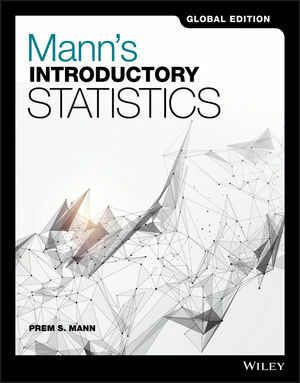# Mann's Introductory Statistics 9th GE Prem Mann 9781119248941

RM 95.00

TITLE : Mann's Introductory Statistics  Prem Mann

ISBN13 : 9781119248941

PUBLISHER : JOHN WILEY (2017)

EDITION : 9TH GLOBAL EDITION PAPERBACK

PAGES : 624 Color Pages

## DESCRIPTION

Mann's Introductory Statistics is written for a one or two semester first course in applied statistics and is intended for students who do not have a strong background in mathematics. The only prerequisite is knowledge of elementary algebra. Introductory Statistics is known for its realistic examples and exercises, clarity and brevity of presentation, and soundness of pedagogical approach.

Table of Content

Chap 1 Introduction

Chap 2 Organizing And Graphing Data

Chap 3 Numerical Descriptive Measures

Chap 4 Probability

Chap 5 Discrete Random Variables And Their Probability Distributions

Chap 6 Continuous Random Variables and The Normal Distribution

Chap 7 Sampling Distributions

Chap 8 Estimation of The Mean and Proportion

Chap 9 Hypothesis Tests About the Mean and Proportion

Chap 10 Estimation And Hypothesis Testing : Two Populations

Chap 11 Chi-Square Tests

Chap 12 Analysis of Variance

Chap 13 Simple Linear Regression

Appendix A Explanation of Data Sets

Appendix B Statistical Tables